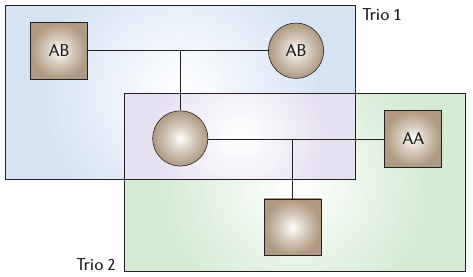August 7, 2014 Statistical Genetics Journal Club• http://sahirbhatnagar.com
• 2nd year PhD student (Biostatistics) with Celia
• Im interested in
• anything applied that can contribute to society
• big data
• reproducible research
• how to give a good talk
• Big believer in open source software

## Disclaimer

1. I will ask alot of questions
3. Your participation is necessary for this to be useful
4. Interrupt me often

## What is Transmission Ratio Distortion (TRD)

• A statistical departure from the Mendelian 1:1 inheritance ratio
• Occurs when one of the two alleles from either parent is preferentially transmitted to the offspring## What is Transmission Ratio Distortion (TRD)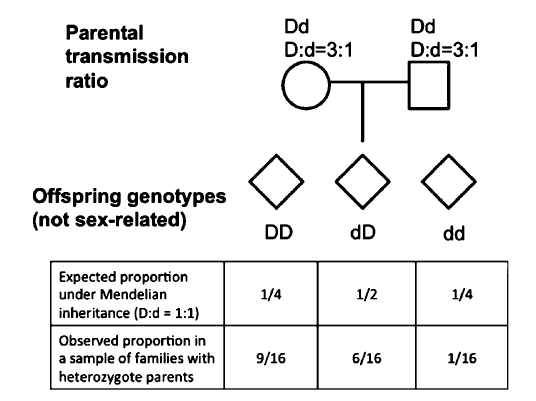## Properties of TRD

• Can act independently of disease status
• there are biological processes that cause TRD
• Extent of TRD and its influence in the human genome remains incomplete
• we didn't find any studies that looked at TRD in WGS data

## Biological Mechanisms of TRD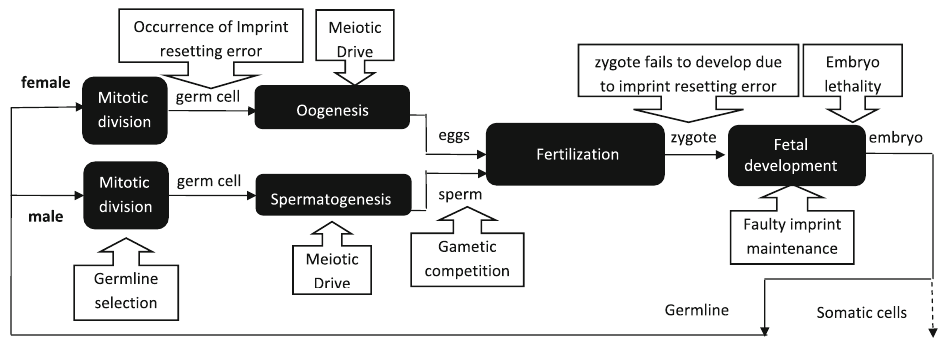## How can we assess TRD ?

### The Transmission Disequilibrium Test (TDT)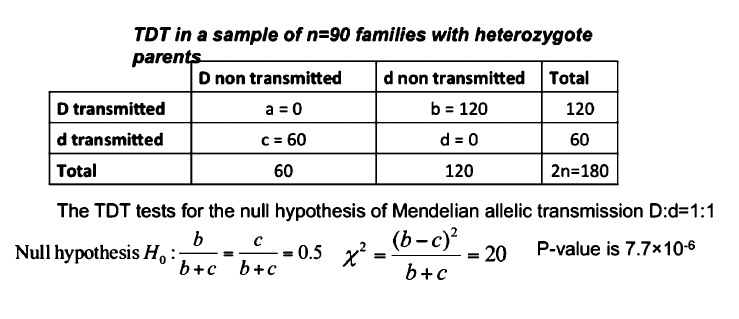## Caveat of assesing TRD

• Can only be observed in family-based studies
• Costs
• Not always feasible to genotype unaffected
• Getting in touch with family members

## Test Statistics

•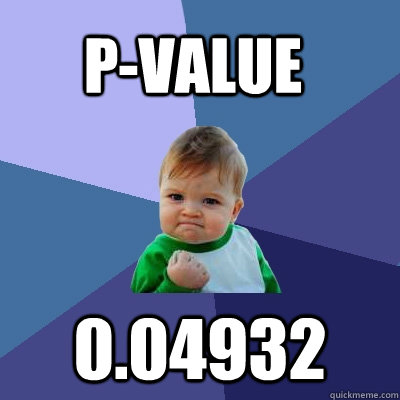## Test Statistics

### What they actually are

- A single measure of a sample
- It reduces the data to one value
- Need to know its sampling distribution to conduct hypothesis tests

$\textrm{Pearson} = \frac{(Observed - Expected)^2}{Expected} \sim \chi^2_{(df)}$

$Z = \frac{\bar{X}-\mu}{\sigma} \sim \mathcal{N}(0,1)$

## Test Statistics

### In general…

$\textrm{Test Statistic} = \frac{\textrm{a measure of deviation from the "truth"}}{\textrm{a scaling factor to account for variability in your sample}}$
• For example:
• $H_0: \textrm{mean height}= 170 cm \rightarrow \textrm{truth''}$
• $\textrm{sample mean } \bar{X}=220cm$
• $\textrm{sample sd } = 60cm$

## What do we mean by Chi-Square Test ?

• Any statistical hypothesis test in which the sampling distribution of the test statistic is a chi-squared distribution when the null hypothesis is true
• Chi-Square Test
• Pearson's goodness of fit
• McNemar's test
• Likelihood ratio test

## Genetic Analysis Workshop 19 (GAW19)

• GAW19 data drawn from T2D-GENES Project
• Whole genome sequencing
• 20 Mexican American Pedigrees from San Antonio
• Enriched for type 2 diabetes
• Genotype calls cleaned of mendelian errors for 959 individuals
• 464 directly sequenced
• 495 imputed
• 8.4 million markers
• odd-numbered autosomes

## Genetic Analysis Workshop 19

### 20 Mexican American Pedigrees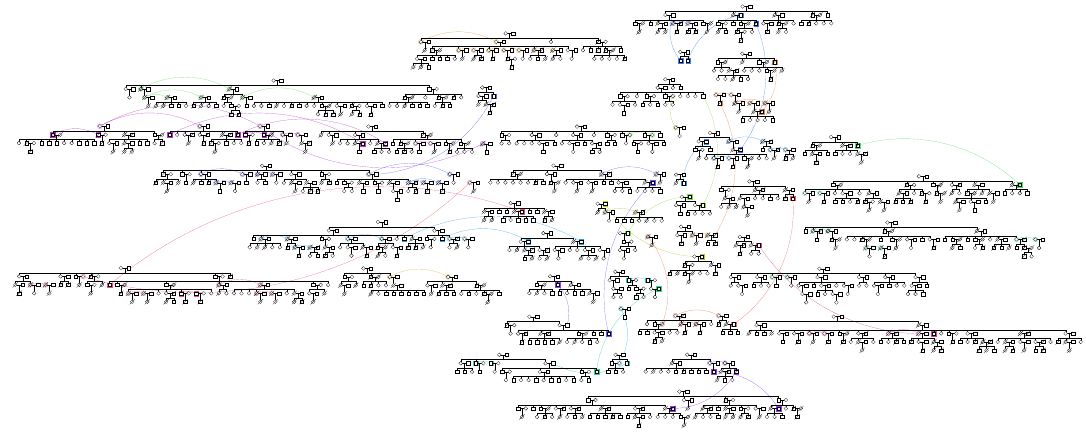## Genetic Analysis Workshop 19

### Imputation Procedure

A novel population-based imputation approach: Prephasing Imputation

1. Haplotypes are estimated for each individual
2. Estimated haplotypes used directly for imputation of sequence variants
3. Imputation ignores family structure
• to improve quality, data was cleaned for mendelian errors
4. For each missing genotype:
• the probabilities of each possible genotype were calculated in the context of the local haplotypes
• the resulting probabilities were then used to generate an appropriately weighted
gene dosage variable

## GAW19 Objective

Identify potentially distorted regions in the genome using family based association methods
•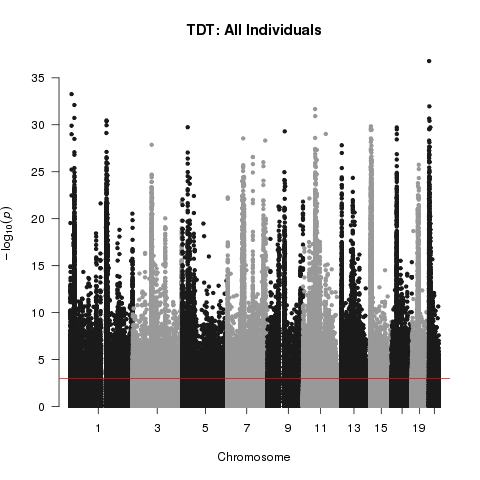## GAW19 Objective

### My Reaction## GAW19 Objective: Take 2

• See if this inflation in TRD $$p$$ values is replicated in other Family Based Tests
• Pedigree Disequilibrium Test (PDT)
• Family Based Association Test (FBAT)
• Compare methods across different subsets of the data
• Everyone (n=1387)
• Sequenced only (n=464)
• 1 Nuclear family per pedigree (n=136)

## Transmission Disequilibrium Test

A non transmitted B non transmitted Total
A transmitted a b a+b
B transmitted c d c+d
Total a+c b+d 2n
• $\chi^2_{(TDT)} = \frac{(b-c)^2}{b+c} \sim \chi^2_{(1)}$
• This is also known as a McNemar Test

## Transmission Disequilibrium Test

### Informative Trios

Need to have at least 1 heterozygous parent for trio to be informative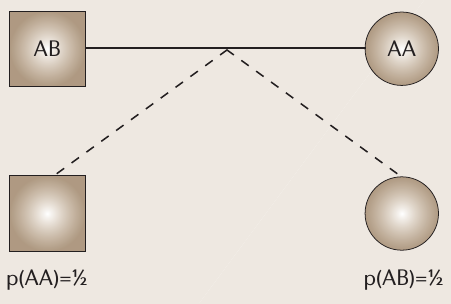## Transmission Disequilibrium Test

### How to assess TRD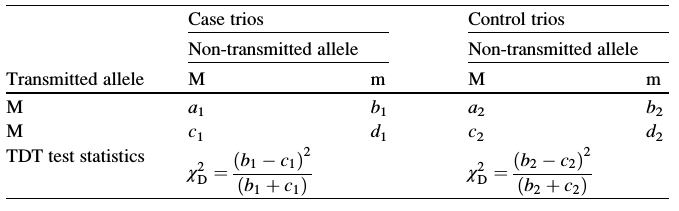## Pedigree Disequilibrium Test (PDT)

Consider a marker with two alleles $$A$$ and $$B$$.

Informative families are:

1. At least one affected child, both parents genotypes, one heterozygous parent
2. Discordant sibships (1 affected, 1 unaffected) with different genotypes, parental genotypes not required

## Pedigree Disequilibrium Test (PDT)

### Informative Trios## Pedigree Disequilibrium Test (PDT)

### Informative Discordant Sibships## Pedigree Disequilibrium Test (PDT)

Within an informative nuclear family define:

• $$X_T$$ = (#$$A$$ transmitted) - (#$$A$$ not transmitted) $$\rightarrow$$ $$n_T$$ trios
• $$X_S$$ = (#$$A$$ affected sib) - (#$$A$$ unaffected sib) $$\rightarrow$$ $$n_s$$ sibships

$D=\frac{1}{n_T + n_S} \left( \sum X_{T} + \sum X_{S} \right)$

For $$k=1,\ldots,N$$ unrelated informative pedigrees

$PDT_{test} = \frac{\sum D_k}{\sqrt{\sum D_k^2 }} \sim \mathcal{N}(0,1)$

## Family Based Association Test (FBAT)

A unified approach to family based tests of association that can handle:
1. Different genetic models
2. Sampling designs
3. Multiallelic markers
4. Quantitative traits
5. Missing parental genotype information

## Family Based Association Test (FBAT)

• The FBAT statistic is based on the covariance between genotype and phenotype
• What is covariance ?
• A measure of how much two random variables change together
• $$R^2$$ is normalized version of the covariance

## Family Based Association Test (FBAT)

### Test Statistic

$U = \sum T^* \left[ X-E(X|P) \right] \rightarrow \textrm{Covariance}$ $FBAT = \frac{U^2}{var(U)} \sim \chi^2_{(1)} \rightarrow \textrm{Test Statistic}$

• $$X$$: translates offspring's genotype to a numeric value e.g. count of A alleles (random)
• $$P$$: genotype of offspring's parents (fixed)
• $$T$$: offspring's trait (fixed)
• summation is over all offspring in the sample

## Family Based Association Test (FBAT)

### Why so flexible ?

• Of interest is the offspring's genotype
• Missing parental genotypes are nuisance parameters
• Standard approach to handling nuisance parameters:
• Find sufficient statistics for them
• Condition on the sufficient statistics
• Conditional distribution does not depend on nuisance parameters

## Family Based Association Test (FBAT)